[BACK]
 Intelligent Automation & Soft ComputingDOI:10.32604/iasc.2021.016884Article

Handwritten Character Recognition Based on Improved Convolutional Neural Network

1School of Computer and Software, Nanjing University of Information Science and Technology, Nanjing, 210044, China
2Jiangsu Key Laboratory of Data Science and Smart Software, Jinling Institute of Technology, Nanjing, 211169, China
3Department of Electronics and Computer Science Koszalin University of Technology Sniadeckich 2, 75-453, Koszalin, Poland
4Faculty of Business, Australian College of Kuwait, State of Kuwait
*Corresponding Author: Yu Xue. E-mail: xueyu@nuist.edu.cn
Received: 14 January 2021; Accepted: 23 March 2021

Abstract: Because of the characteristics of high redundancy, high parallelism and nonlinearity in the handwritten character recognition model, the convolutional neural networks (CNNs) are becoming the first choice to solve these complex problems. The complexity, the types of characters, the character similarity of the handwritten character dataset, and the choice of optimizers all have a great impact on the network model, resulting in low accuracy, high loss, and other problems. In view of the existence of these problems, an improved LeNet-5 model is proposed. Through increasing its convolutional layers and fully connected layers, higher quality features can be extracted. Secondly, a more complex dataset called EMNIST is selected and many experiments are carried out. After many experiments, the Adam optimization algorithm is finally chosen to optimize the network model. Then, for processing character similarity problems on the pre-processed EMNIST dataset, the dataset is divided into different parts and to be processed. A better-divided result is selected after the comparative experiments. Finally, the high accuracy recognition of handwritten characters is achieved. The experimental results show that the recognition accuracy of the handwritten characters reached at 88% in the test set, and the loss is low.

Keywords: Convolutional neural networks; handwritten character recognition; tensorflow; optimizer

1  Introduction

In this era of big data, there are a great number of text data that need to be processed. These data are closely related to people's lives. Such as signs everywhere on the street, product signs in daily life, and data that need to be processed by the staff in daily work. Therefore, to adapt to the development trend of the age of science and technology, or to free people from such complicated work has become more important. It is necessary to realize the automatic detection, the recognition, and the preservation of a great number of handwritten characters quickly and accurately. To solve these problems, researchers began to engage in character recognition research, thus promoting the emergence and development of optical character recognition (ORC) technology. The development of ORC technology can be traced back to the early of 19th century, and it was not formally proposed until 1928 .

ORC is the real-time and efficient positioning and recognition of text information in pictures. ORC can recognize various characters and text information in different scenes. Number recognition is relatively simple and easy to be recognized. However, due to the similarity of characters and the complexity of the dataset, there are certain challenges and difficulties in the recognition of 52 uppercase and lowercase letters . Text recognition, especially because of the different glyphs, whose structure presents high complexity, needs to recognize up to thousands of characters . It is difficult to identify these characters quickly. The existing character similarity problem and a large amount of abnormal data in the dataset must also be solved. There are currently some methods that can be used to optimize ORC technology, such as adaptive feature selection algorithm and particle swarm algorithm .

In the research process, well-known researchers constantly innovate algorithms. These innovative concepts promote the following development of handwritten recognition technology and promote its rapid development . At the same time, these innovative thoughts and algorithms are also applicable in other fields, and correspondingly promote the vigorous development of other industries. From the point of view of the research status, there are various character recognition algorithms at the present stage, both in terms of recognition accuracy and speed. They all have achieved good results . At present, character recognition based on neural networks is much better than some traditional algorithms. Therefore, this paper proposes an improved CNN model, which is trained on a dataset containing numbers and uppercase and lowercase characters. Finally, we realize handwritten character recognition .

The main contributions of this paper are as follows:

1.   A more reasonable division of the dataset is used to avoid the influence of character similarity on experimental accuracy. Experimental results show that this method effectively alleviates the problems caused by character similarity.

2.   An improved LeNet-5 network model is proposed. Compared with the original model, by increasing its number of layers, it is found that it has better performance. Under the same dataset, the accuracy of this model is improved by about 4% compared with the original LeNet-5 network model.

3.   The Adam optimizer, which is more suitable for this experiment is adopted. Through comparing the influence of the current commonly used optimizer on the experimental results and doing a great number of experiments, Adam optimizer is finally adopted, with an accuracy increase of 6% compared with SGD optimizer.

4.   We implemented this experiment on a more complex dataset named EMNIST, and currently there are not many people doing experiments on this dataset based on LeNet-5.

2  Related Work

2.1 LeNet-5 Model

LeNet-5 is often used in character recognition tasks and has achieved good results so far. In this paper, LeNet-5 is trained on the dataset. The network structure of LeNet-5 consists of seven kinds of layers, mainly including the input layer, output layer, convolutional layers, pooling layers, and fully connected layers. The input of the latter layer is the output of the former layer . In addition to the input layer, every other layer has trainable parameters, and each layer has more than one feature map. Each feature map uses a filter to extract the effective features of the input image, and then each Feature Map has more than one neuron. The LeNet-5 structure is shown in Fig. 1 below. It is the first CNN which is applied to digital recognition successfully .

The function of the convolutional layer is to extract the effective features of the input image information. When a character image is fed into the input layer, some information about the image is useful to the model, but some information is useless. If the information of the entire image is input into the neural network for processing, the useless information not only affects the training speed, but also leads to low accuracy. Therefore, after being processed by the convolutional layer filter, the effective features in this picture will be extracted. Then these messages will be input into the neural network, which will be conducive to the training of the neural network, and a better network model will be obtained.

The convolution operation of the convolutional layer is accomplished by using filters. The function of the filters is to transform an input child node matrix on the current layer neural network into a unit child node matrix on the next layer neural network by using the filters and input node matrix to do the element-wise product operation . The filters and the input matrix with the same size as the filters do the element-wise product operation. Then, we move a filter from top to bottom, from left to right under a certain step size, constantly do the element-wise product operation. Finally, we will get a dimensionless node matrix.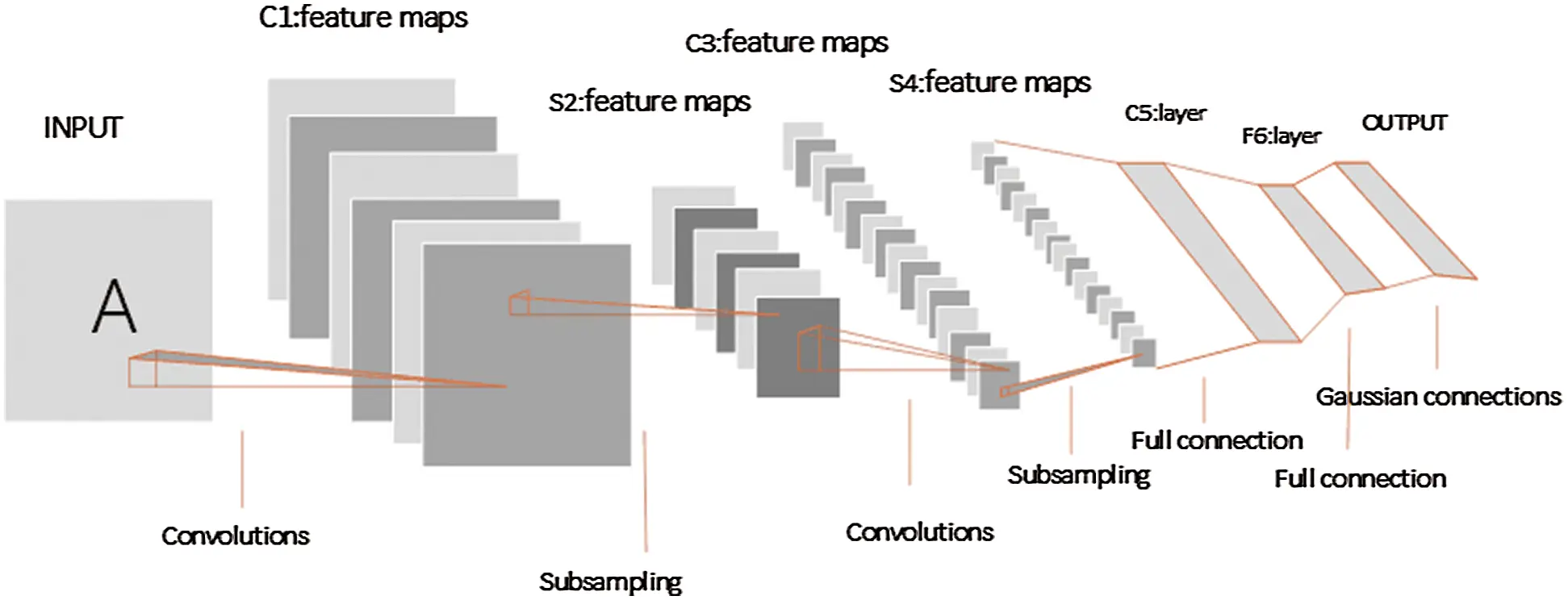Figure 1: LeNet-5 model structure diagram

To better explain the convolution operation process of the convolution layer, a specific example is introduced next. In the subsection, we need to convert a 7 × 7 × 3 input node matrix after processing through two 3 × 3 × 3 filters into a 3 × 3 × 2 output node matrix with a moving step of 2. In this paper, we use wx,y,zi to represent the node number i of the whole weight of input matrix, use bi to represent the bias parameter of the input node number i, use ax,y,z to represent the value of the filter node number (x, y, z). The length of the node matrix is x, and the width is y. The depth is z, which is the number of channels. Therefore, the value g(i) of the output node number i from the node matrix is as follows:

g(i)=f(x=17y=17z=13ax,y,z×wix,y,z+bi) (1)

Like convolution, pooling of some parameters of the filters are set in advance. But different with element-wise product of the convolutional layers, the filter operation of the pooling layer is an operation to calculate the maximum or average value of the pooling area, which can be divided into the max pooling and the average pooling. The advantages of the pooling layer are that it can improve the operation speed of the whole model, reduce the number of parameters of the fully connected layers, and prevent the occurrence of overfitting. Therefore, the pooling layer is a relatively important structure. Pooling operation reduces the size of the image but does not change the number of feature maps. The pooling filters also traverses from left to right and from top to bottom in accordance with a certain step size. The max or average value of the pooling area of the same size is calculated and the result is output.

The max pooling operation equation is as follows:

pl=maxicci (2)

The average pooling operation equation is as follows:

pl=1nicci (3)

i is the node number i of the input node matrix, ci represents the value of the node number i, and n represents the number of nodes.

After all the input features are sorted out and calculated, a fully connected layer turns them into a one-dimensional array, which corresponds to the sample space. In other words, the fully connected layer finally classifies the extracted features. The neurons in a fully connected layer connected with the neurons in the previous layer and the neurons in the latter layer. Meanwhile, the fully connected layers can also integrate the characteristic information in the convolutional layers and pooling layers. Therefore, compared with the convolutional layers and the pooling layers, the fully connected layers have a very large number of parameters. In order to solve the problem of too many parameters, the dropout operation  is used, which can randomly deactivate some parts of the neurons in the neural network, so that this problem can be solved. In general, every neuron in the fully connected layers uses the rectified linear unit (ReLU) as the excitation function to improve the performance of the model.

2.2 Improved LeNet-5 Network Model

This paper initially used LeNet-5 network model to be trained on the dataset. In order to improve the accuracy and the generalization ability of this model, so in this paper, the LeNet-5 has been improved. The improvements in LeNet-5 are shown in Tab. 1 below. It has been proved that the training accuracy and the generalization ability of the improved network model all have been improved.

Table 1: The structure of Improved LeNet-5 network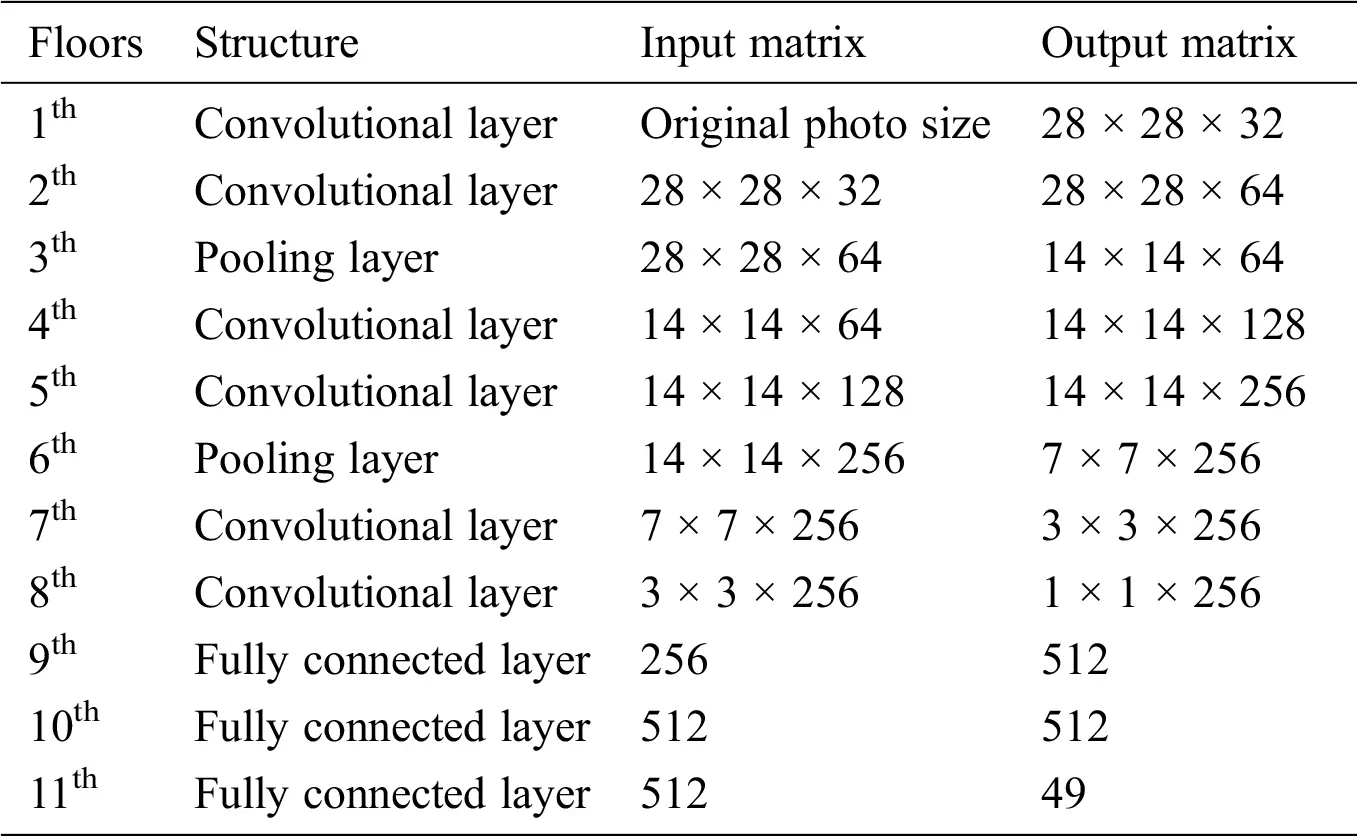2.3 Handwritten Character Recognition Process

The process of handwritten character recognition based on the convolutional neural networks can be divided into four stages.

The first stage is the data preprocessing stage, in which the main task is to preprocess the data image, including the cutting and standardization of the image data, and then convert its format into the format required by the neural network input. Divide the training set and test set, then extract the characteristics of the data, and finally make the image data into memory object dataset.

The second stage is the model construction stage, in which the main task is to determine the convolutional neural network model to be used, to calculate the dimension and size of each parameter, and then set the hyperparameter of the model. Finally, python language is used to build the model on the TensorFlow framework.

Then, in the training stage, in which the constructed neural network model is trained on the pre-processed training dataset, and the model is saved every 1,000 rounds. The graph of its accuracy changing with the number of iterations is printed to observe the change of its accuracy.

After that, in the validation phase, it is used to assess the model stage. According to the results of the validation dataset, we adjust the network structure and control the parameters of the model complexity to achieve the optimal model on the validation dataset. Then, we print graphs of accuracy and loss that vary with the number of iterations, and observe the changes of its accuracy and loss.

Finally, there is the test phase. This phase tests the model that performs best in the verification phase on the test dataset. It can also predict the characters in a single image and observe whether the model can recognize the characters correctly.

2.4 Introduction to Dataset

In this paper, EMNIST  dataset which is very similar to the MNIST dataset is selected, and the quantity, category, and exception of the dataset are processed accordingly. Finally, the network model in this paper is trained and tested on this dataset.

EMNIST is known as MNIST. However, the accuracy of most network models in the MNIST dataset is excellent, so on this basis, the EMNIST dataset is launched, and the image size and interface of this dataset are like MNIST. As the EMNIST dataset is a preprocessed dataset that can be directly used in the network model, there is no need for normalization, centralization, binarization, refinement, and other operations.

As the EMNIST dataset is a foreign dataset, its character writing mode is different from the traditional Chinese writing mode. They usually like to use the connecting pen, and these data are not filtered, so the dataset contains some abnormal data that is difficult to be recognized, as shown in Fig. 2. According to the causes of data exceptions, abnormal data can be roughly divided into the following types:

1) The writing character is too small or the connecting pen makes it unrecognizable, which affects the recognition accuracy.

2) The strokes of writing characters are too vague to be recognized, resulting in the unknown shape of the characters.

3) Due to different writing habits, the characters seem so similar to other types of characters.

4) The differences between different characters are too small to distinguish.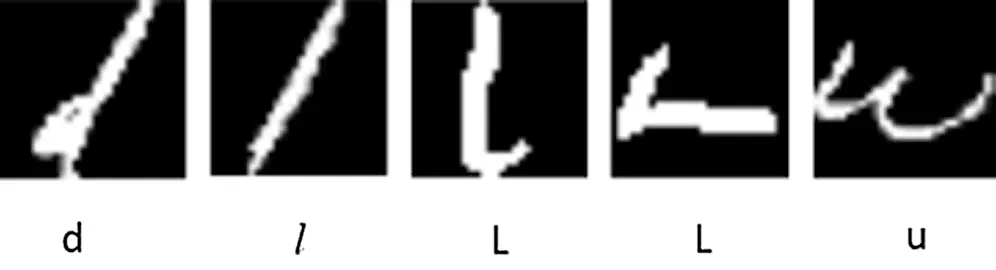Figure 2: Example of abnormal data

These abnormal data will affect the experimental accuracy and cause unnecessary errors. In order to avoid the affection of abnormal data, this paper deletes the data with the exception problems. All data in the dataset are standardized.

3  Experiment and Result Analysis

3.1 Experimental Data Processing

In order to alleviate the influence of character similarity on the result, a new partition of the dataset is made in this paper to solve the influence. The model is trained and validated on three sets of data with different results, and the experimental results are analyzed to compare which dataset is more suitable for this experiment.

1) The first dataset consists of 62 characters, including numeric characters 0 to 9, uppercase and lowercase of English letters.

2) The second dataset consists of 36 characters. Uppercase and lowercase letters are grouped together, and the influence on the experimental results is observed to verify the existence of capitalization and letter similarity.

3) The third dataset contains 49 characters. Based on the first dataset, the similar characters (0, o, O), (Z, z), (1, L), (P, p), (X, x), (S, s), (W, w), (U, u), (V, v), (M, m), (K, k), (C, c) are classified and processed, i.e., (0, o, O) are classified into one class, and so are the others.

The specific categories of the datasets are used in this paper and the corresponding number of training dataset, verification dataset, and test dataset is shown in Tab. 2.

Table 2: Datasets division information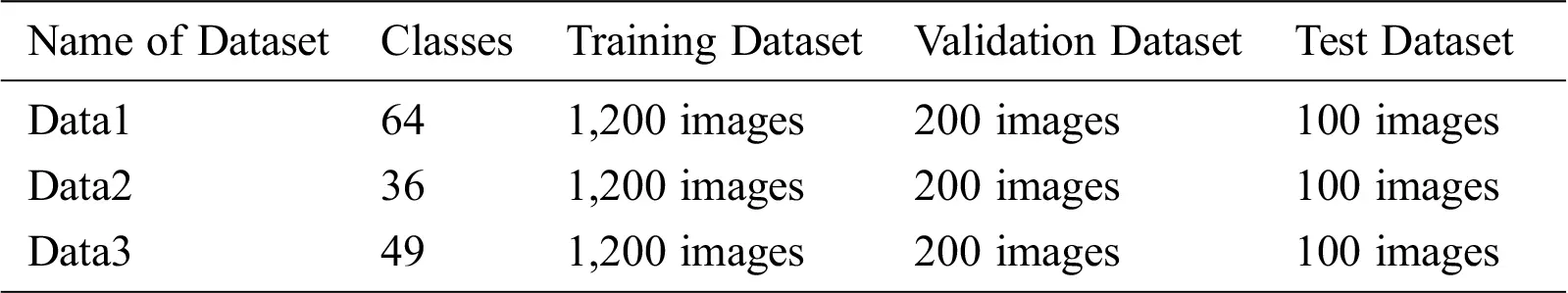3.2 Experimental Parameter Setting

The basic learning rate used in this paper is 0.8, and the learning rate decay rate is 0.99. The exponential decay method is adopted to control the change of the learning rate. The number of training rounds is 100,000, and the training model is saved every 1,000 rounds. In order to prevent overfitting during the training, the dropout method is used in this paper. In the process of calculating the loss value, L2 regularization is used to improve the fitting .

In this paper, 1,200 pictures/classes are used as training data, 200 pictures/classes as verification data, and 100 pictures/classes as test data.

3.3 Evaluation Index Parameters

Accuracy: The prediction is made on the network model with the data. If the real information of the data is consistent with the predicted information, the prediction is correct. Usually, the output of the neural network is a vector, and each dimension of the vector represents the probability of a certain category the data is. The number of categories corresponds to the number of probability values, and then the maximum value is taken as the predicted value. Accuracy is the percentage of correctly predicted data in total data of a batch of datasets .

Loss: It is used to measure the consistency between the output value of the neural network and the true value of the sample .

3.4 The Experiment Platform

The experiments in this paper are all run on Windows (64bit) system, and the training and testing software of the deep learning neural network framework is TensorFlow . The running memory of CPU is 16GB and the GPU is one GTX1080Ti.

4  Analysis of Experimental Results

4.1 A Comparative Experiment on Character

During the training on the 62 characters (including numbers and uppercase and lowercase letters), the accuracy is not very good, only around 70%. The shock of loss is huge. Therefore, this paper begins to try to deal with some categories that are too similar in order to improve the recognition accuracy of the network model .

Therefore, this paper trains the improved LeNet-5 network model on three different datasets and verifies it. Finally, the experimental results are analyzed and the most suitable dataset is chosen for this experiment [25,26].

The network model is trained on the first set of data and verified on the validation set, and its verification accuracy is shown in Fig. 3.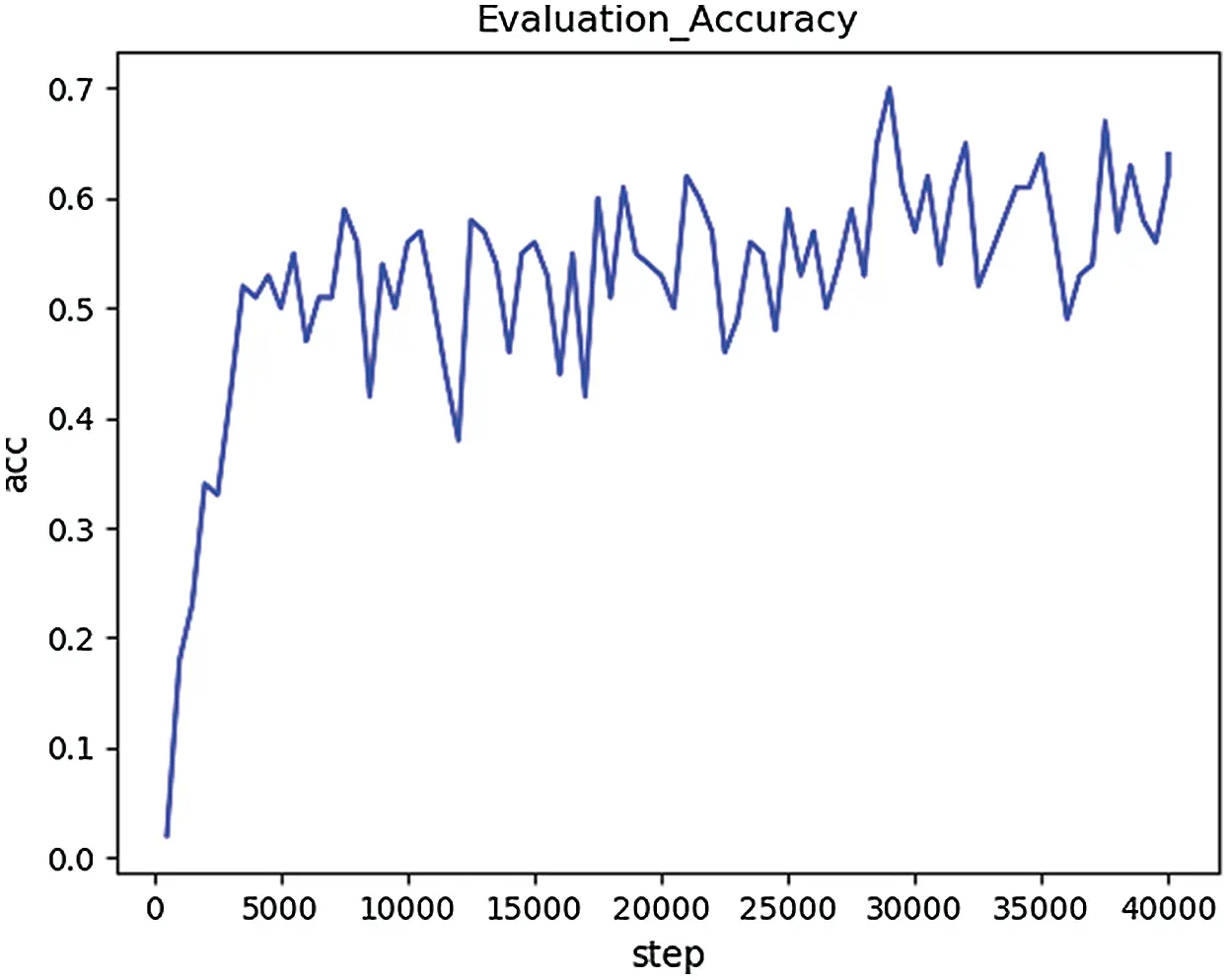Figure 3: The validation accuracy based on Data1

The network model is trained on the second dataset and verified on the validation set, and its verification accuracy is shown in Fig. 4.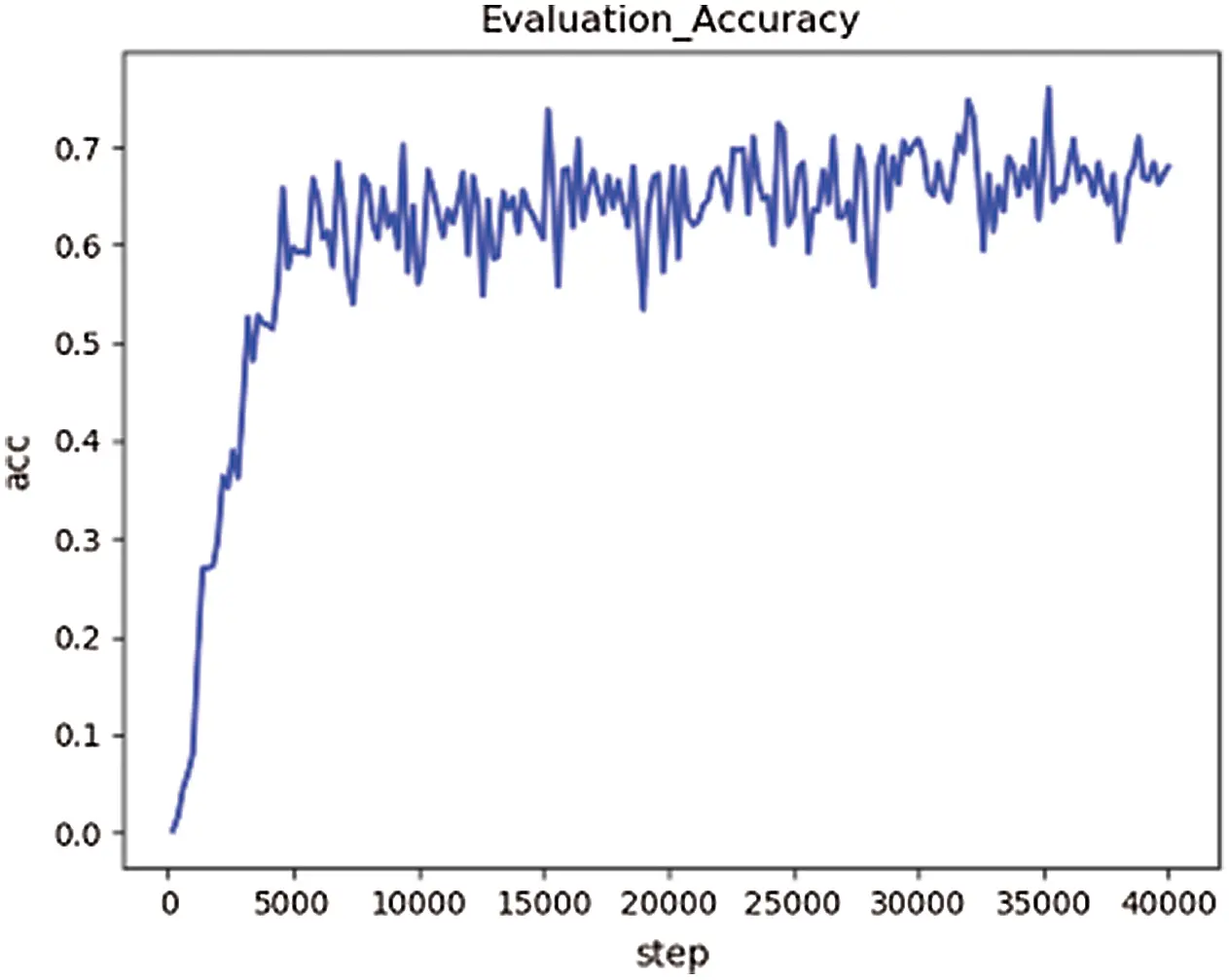Figure 4: The validation accuracy based on Data2

The network model is trained on the third dataset and verified on the validation set, and the verification accuracy is shown in Fig. 5.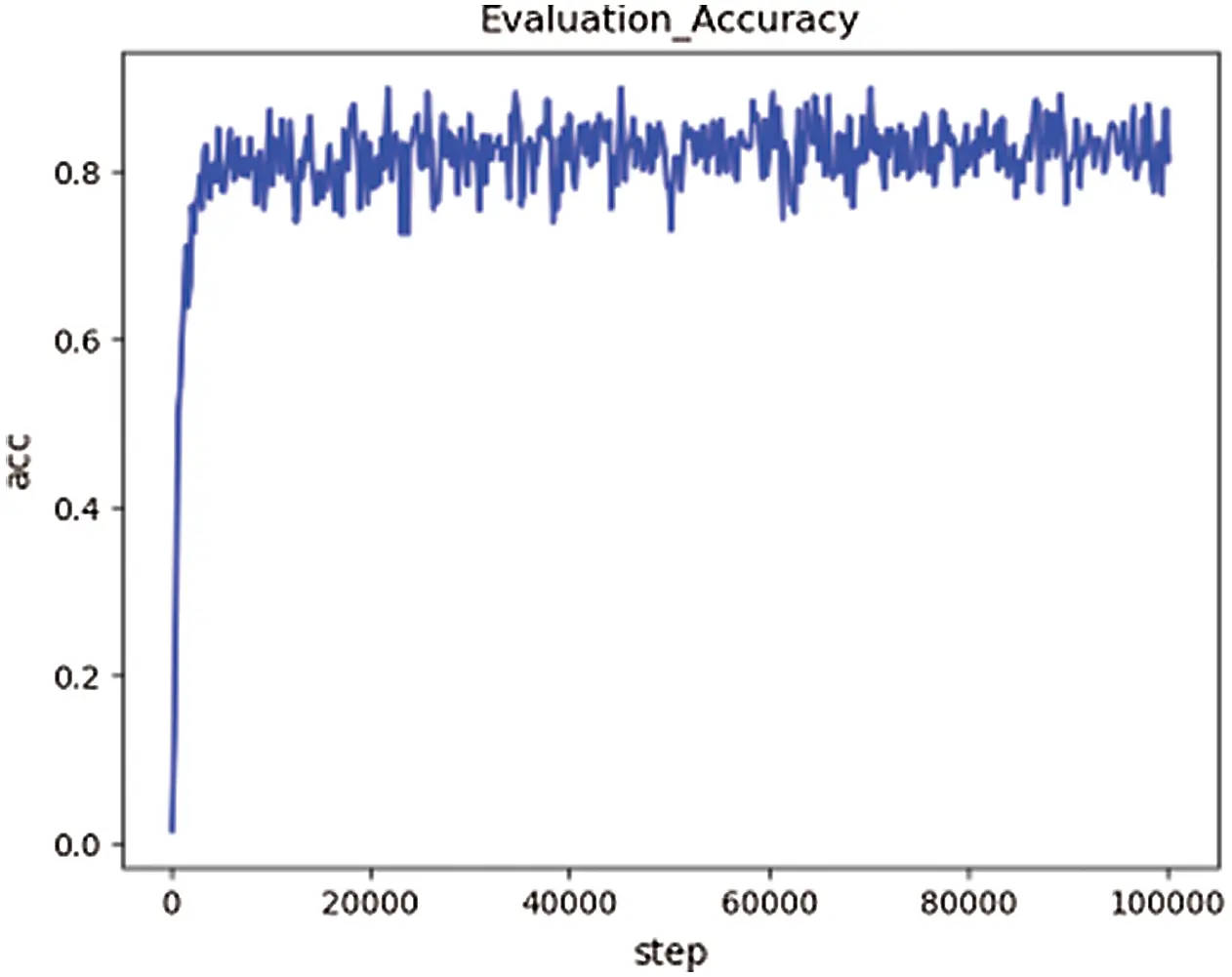Figure 5: The validation accuracy based on Data3

The improved LeNet-5 network model is used to train on the three datasets respectively, and verification is carried out on the verification set. The comparison results of verification accuracy are shown in Fig. 6.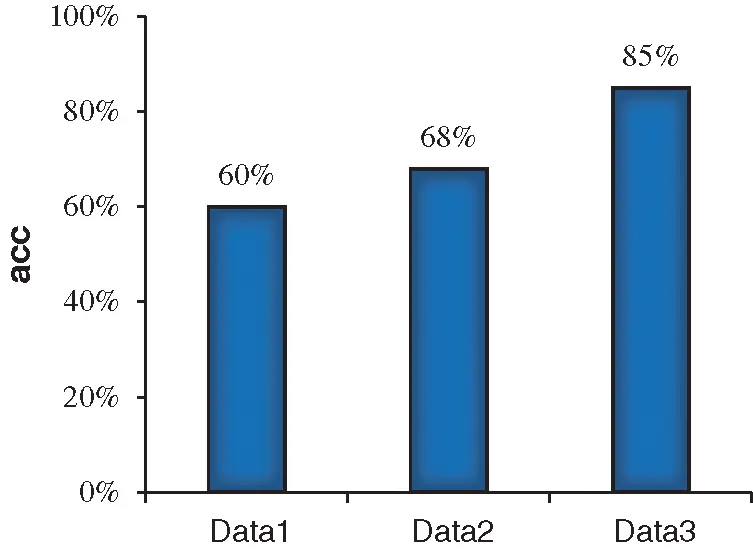Figure 6: Comparison of verification accuracy on the three datasets

According to the experimental results, the accuracy of the training sets of the above three groups is close to 98% after 40,000 rounds of training (overfitting problem exists). However, when verifying the model, the verification accuracy obtained on the first dataset is the lowest, about 60%. After training and verification on the second dataset, the verification accuracy is about 68%, which is 8% higher than that of the first group of experiments. In the third group of experiments, the verification accuracy rate is about 85%, which has reached a good accuracy.

Therefore, it can be concluded that the third dataset after character similarity classification has a better result.

4.2 Model Performance Comparison

In order to verify the effectiveness of the improved LeNet-5 model, the performance of the LeNet-5 model under the same dataset is compared. It has been proved that with the improved network model, both the training accuracy and the generalization ability of the model have been improved . As shown in Fig. 7, the accuracy has been improved by about 0.3.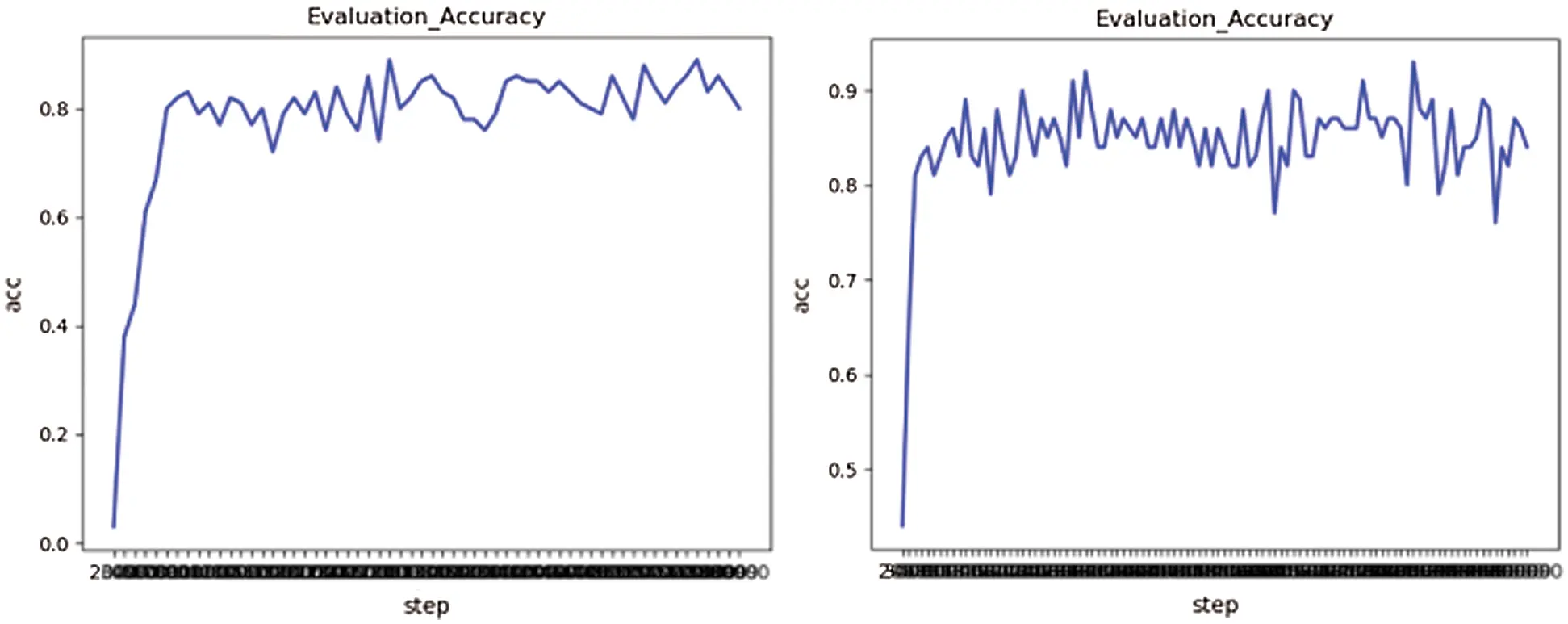Figure 7: Verifies that the accuracy changes with the number of iterations, with the picture on the left of the experiment on LeNet-5 and the picture on the right of the actual improvement model

On the Data3, the improved LeNet-5 model is to be trained on the training dataset. The number of training rounds was 100,000, and the model was saved every 1,000 rounds. The model that has been saved during the training is verified on the validation dataset, as shown in Fig. 8. The accuracy of verification is about 85%.Figure 8: The accuracy of verification

In order to get better results on the test set, a model with the optimal performance on the verification dataset should be found, as shown in Fig. 9. The figure shows the accuracy of the verification set for different iteration times. We test the model that performs best on the validation dataset on the test dataset, and print training accuracy and loss every 1,000 times.

As shown in Fig. 10, the test accuracy of this model has reached about 88%, which still needs to be improved, but it still achieves a relatively good result.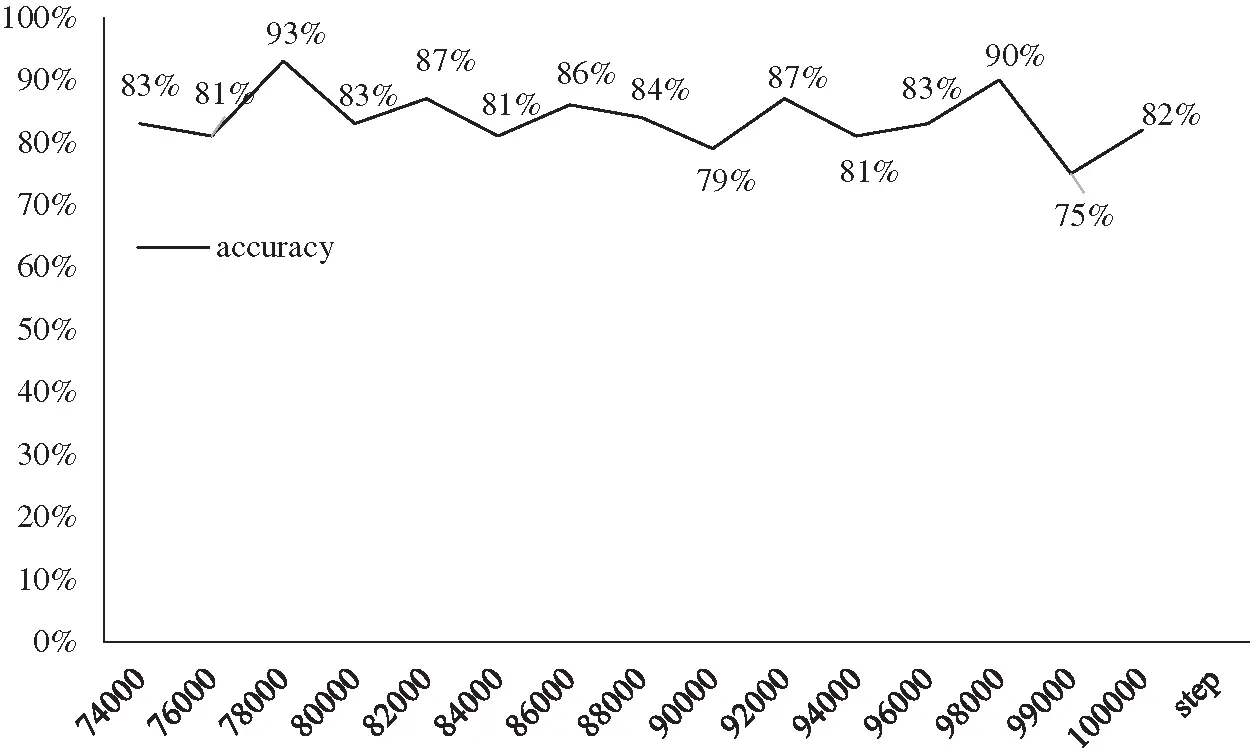Figure 9: The accuracy on the verification dataset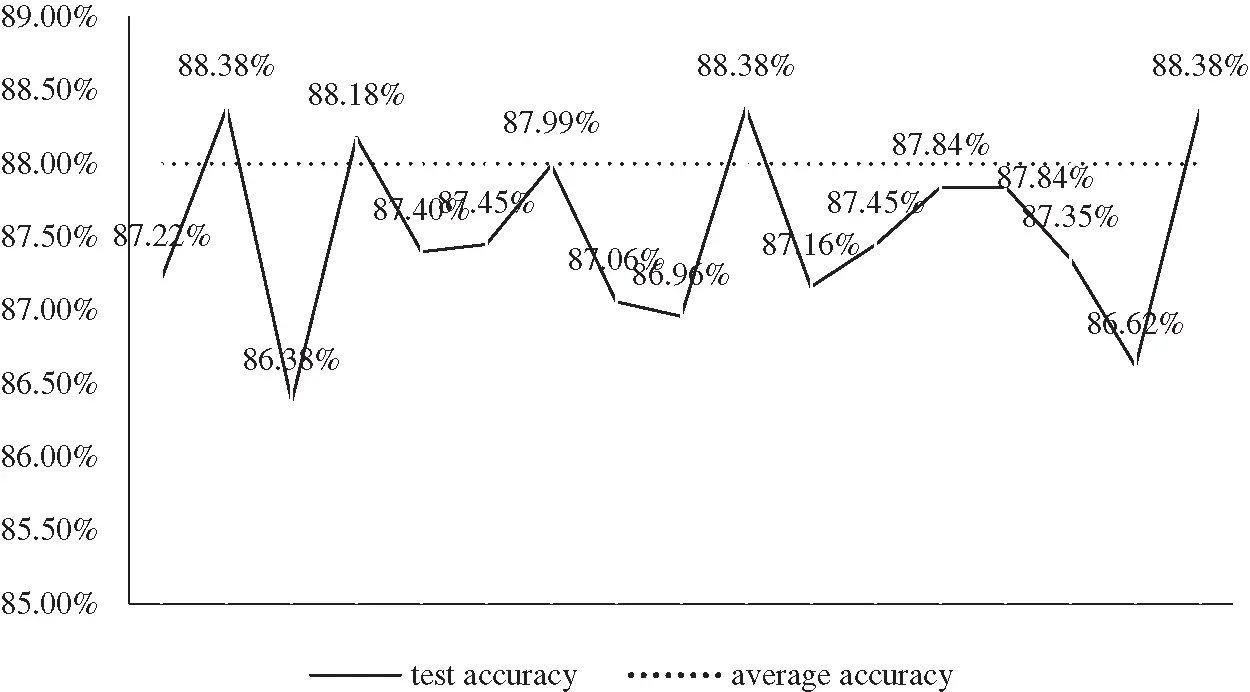Figure 10: This is a functional performance based on test set

4.3 Optimizer Performance Comparison

The choice of the optimizer has a great impact on the model, and different optimization algorithms will produce different effects in the same experiment. Although the gradient descent algorithm is the most widely used one at present, many different optimization algorithms have appeared at present [30,31]. Therefore, this section will try to use other optimizers to optimize the model. Based on the training of 50,000 rounds, it will observe the changes in the accuracy of the test dataset by optimizing the network model with several other commonly used optimizers, to find a more suitable optimizer for handwritten character recognition and improve the recognition accuracy. Fig. 11 shows the effect of the optimization model with different optimizers on the validation set.Figure 11: Performance comparison of different optimizers

4.4 Recognition of Individual Characters

To use the trained model to predict the characters in a single image, the input image should be preprocessed first, including image clipping, standardization, and changing the image format as [1,28,28,1]. The picture to be predicted is shown in Fig. 12. 14 is the label of the picture, indicating that the picture belongs to category ‘g’. The expected predicted value should be 14.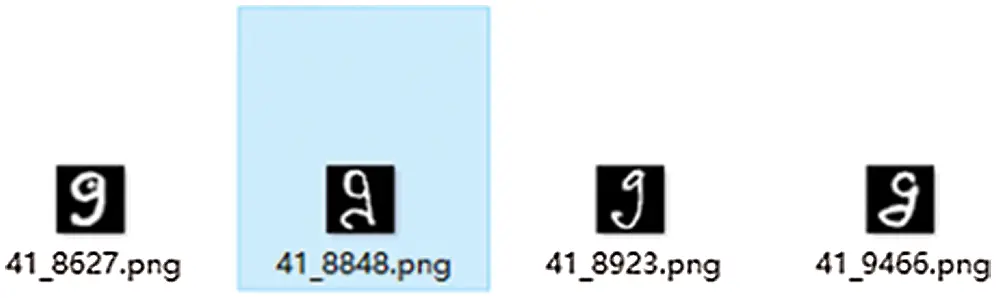Figure 12: The samples to be predicted

After this character is recognized by the network model, the predicted value and the true value can be output. In the experiment, the predicted value is 14. The predicted value is the same as the real value and the prediction is correct.

5  Conclusions

Today, the accuracy and efficiency of handwritten digit recognition have reached a high level. Its recognition rate has reached nearly 100%. Therefore, based on handwritten digit recognition, this paper first challenges more datasets of handwritten character types and improves the LeNet-5 convolutional neural network model, which is proved to be more expressive in this experiment. Secondly, apply different classification processes to the dataset, and verify the influence of similarity on accuracy based on experiments to select a better classification effect. In addition, different optimizers are used to compare the influence on the experimental results, so as to adopt a more suitable optimization algorithm. Finally, combined with the TensorFlow framework, handwritten character recognition was realized, and the recognition rate reached 88%.

Funding Statement: This work was partially supported by the National Natural Science Foundation of China (61876089, 61876185, 61902281, 61375121), the Opening Project of Jiangsu Key Laboratory of Data Science and Smart Software (No.2019DS301), the Engineering Research Center of Digital Forensics, Ministry of Education, the Key Research and Development Program of Nanjing Jiangbei New Area (ZDYF20200129), and the Priority Academic Program Development of Jiangsu Higher Education Institutions.

Conflicts of Interest: The authors declare that they have no conflicts of interest to report regarding the present study.

## References

1. S. Wang, “Handwritten character recognition based on convolutional neural network,” Chang'an University, 2019.
2. Y. Xue, J. M. Jiang, B. P. Zhao and T. H. Ma, “A self-adaptive artificial bee colony algorithm based on global best for global optimization,” Soft Computing, vol. 22, no. 9, pp. 2935–2952, 2018.
3. Y. Xue, T. Tang, W. Pang and A. X. Liu, “Self-adaptive parameter and strategy based on particle swarm optimization for large-scale feature selection problems with multiple classifiers,” Applied Soft Computing, vol. 88, no. 4, pp. 106031, 2020.
4.    Y. Xue, B. Xue and M. Zhang, “Self-adaptive particle swarm optimization for large-scale feature selection in classification,” ACM Transactions on Knowledge Discovery from Data, vol. 13, no. 5, pp. 1–27, 2019.
5.    X. Yu, Y. Chu, F. Jiang, Y. Guo and D. W. Gong, “SVMs classification based two-side cross domain collaborative filtering by inferring intrinsic user and item features,” Knowledge-Based Systems, vol. 141, no. 1, pp. 80–91, 2018.
6.    Y. Zhang, X. F. Song and D. W. Gong, “A return-cost-based binary firefly algorithm for feature selection,” Information Sciences, vol. 418-419, no. 3, pp. 561–574, 2017.
7. Y. Zhang, D. W. Gong, Y. Hu and W. Q. Zhang, “Feature selection algorithm based on bare bones particle swarm optimization,” Neurocomputing, vol. 148, no. 5, pp. 150–157, 2015.
8. S. Cheng and Y. H. Shi, “Brain storm optimization algorithms: Concepts, principles and applications,” Adaptation, Learning, and Optimization. Vol. 23. Springer International Publishing AG, 2019.
9. S. Cheng, L. B. Ma, H. Lu, X. J. Lei and Y. H. Shi, “Evolutionary computation for solving search-based data analytics problems,” Artificial Intelligence Review, in press, 2020.
10. Y. Zhang, D. W. Gong and J. Cheng, “Multi-objective particle swarm optimization approach for cost-based feature selection in classification,” IEEE/ACM Transactions on Computational Biology and Bioinformatics, vol. 14, no. 1, pp. 64–75, 2017.
11. L. B. Ma, S. Cheng and Y. H. Shi, “Enhancing learning efficiency of brain storm optimization via orthogonal learning design, ” IEEE Transactions on Systems. Man, and Cybernetics: Systems, 2020.
12. B. Cao, J. W. Zhao, P. Yang, Y. Gu, Khan Muhammad et al., “Multi-objective 3-D topology optimization of next-generation wireless data center network,” IEEE Transactions on Industrial Informatics, vol. 16, no. 5, pp. 3597–3605, 2020.
13. A. J. Qi, “Talk about text recognition software OCR,” Printing Technology, vol. 000, no. 013, pp. 27–30, 2004.
14. Z. W. Zhang, “Study of CNN and the application in character recognition,” Liaoning University of Science and Technology, 2018.
15. Q. C. Zhou, X. C. Liu, H. Zhao, H. H. Shen and X. L. Xiong, “Fault diagnosis for rotating machinery based on 1D depth convolutional neural network,” Vibration and Impact, vol. 37, no. 23, pp. 39–45, 2018.
16. Y. Ju, X. Wang and X. Chen, “Research on OMR recognition based on convolutional neural network tensorflow platform,” in 2019 11th Int. Conf. on Measuring Technology and Mechatronics Automation (ICMTMAQiqihar, China, pp. 688–691, 2019.
17. Z. Y. Zhen and S. Y. Gu, “TensorFlow: Real google deep learning framework,” Beijing: Electronic Industry Press, 67–86,20
18. G. H. Dong, “Research on human action recognition base on deep learning,” Xi’an University of Posts, 20
19. Cohen, Gregory, Afshar, Saeed, Tapson et al., “EMNIST: An extension of MNIST to handwritten letters,” 2017.
20. M. G. Guo and H. Gong, “Optimization of convolutional neural network based on tensorflow,” CEA, vol. 56, no. 1, pp. 158, 20
21. Y. F. Liu, “Study on the application of traffic image recognition based on reel neural networks,” Chang'an University, 2018.
22. C. Qian, “Research on deep learning based on face recognition,” Southwest Jiaotong University, 2017.
23. Tomomi Ishida, “Semantic ambiguity effects in L2 word recognition,” Journal of Psycholinguistic Research, vol. 47, no. 3, pp. 523–536, 2018.
24. Z. X. Sun, “TensorFlow-based handwriting digital identification,” Changchun Polytechnic University, 2019.
25. L. Ma, X. S. Luo and P. Q. Jiang, “Research on dot matrix character recognition based on template matching and support vector,” Computer Engineering and Applications, vol. 56, no. 4, pp. 134–139, 2020.
26. C. Y. Qin, P. Zheng and X. Zhang, “Offline handwritten chinese character recognition based on MQDF-DBM model,” Computer Engineering and Applications, vol. 56, no. 7, pp. 141–146, 2020.
27. A. Feng, Z. Gao, X. Song, K. Ke, T. Xu et al., “Modeling multi-targets sentiment classification via graph convolutional networks and auxiliary relation,” Computers, Materials & Continua, vol. 64, no. 2, pp. 909–923, 2020.
28. C. Anitescu, E. Atroshchenko, N. Alajlan and T. Rabczuk, “Artificial neural network methods for the solution of second order boundary value problems,” Computers, Materials & Continua, vol. 59, no. 1, pp. 345–359, 2019.
29. C. Hung, W. L. Mao and H. Y. Huang, “Modified PSO algorithm on recurrent fuzzy neural network for system identification,” Intelligent Automation & Soft Computing, vol. 25, no. 2, pp. 329–341, 2019.
30. F. Zhang, H. Zhao, W. Ying, Q. Liu, A. Noel et al., “Human face sketch to RGB image with edge optimization and generative adversarial networks,” Intelligent Automation & Soft Computing, vol. 26, no. 4, pp. 1391–1401, 2020.
31. H. Zhou, Y. J. Chen and S. M. Zhang, “Ship trajectory prediction based on BP neural network,” Journal on Artificial Intelligence, vol. 1, no. 1, pp. 29–36, 2019.This work is licensed under a Creative Commons Attribution 4.0 International License, which permits unrestricted use, distribution, and reproduction in any medium, provided the original work is properly cited.